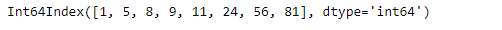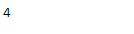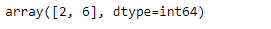# Python | Pandas Index.searchsorted()

Python is a great language for doing data analysis, primarily because of the fantastic ecosystem of data-centric python packages. Pandas is one of those packages and makes importing and analyzing data much easier.

Pandas` Index.searchsorted()` function find indices where elements should be inserted to maintain order. The function find the indices into a sorted IndexOpsMixin self such that, if the corresponding elements in value were inserted before the indices, the order of self would be preserved.

Syntax: Index.searchsorted(value, side=’left’, sorter=None)

Parameters :
value : Values to insert into self.
side : If ‘left’, the index of the first suitable location found is given. If ‘right’, return the last such index. If there is no suitable index, return either 0 or N (where N is the length of self).
sorter : Optional array of integer indices that sort self into ascending order. They are typically the result of np.argsort.

Returns : [indices : array of ints] Array of insertion points with the same shape as value.

Example #1: Use `Index.searchsorted()` function to find the correct position to insert an element such that the Index remains sorted.

 `# importing pandas as pd ` `import` `pandas as pd ` ` `  `# Creating the index ` `idx ``=` `pd.Index([``1``, ``5``, ``8``, ``9``, ``11``, ``24``, ``56``, ``81``]) ` ` `  `# Print the Index ` `idx `

Output :Let’s find the position of for insertion if the element to be inserted is 10

 `# to find the position of inseretion ` `idx.searchsorted(``10``) `

Output :As we can see in the output, the function has returned 4 indicating that the correct position to insert 10 in the index is 4 if the order is to be maintained.

Example #2: Use `Index.searchsorted()` function to find the correct position of insertion for more than one elements in the Index. The insertion should be done such that the order is maintained.

 `# importing pandas as pd ` `import` `pandas as pd ` ` `  `# Creating the index ` `idx ``=` `pd.Index([``1``, ``5``, ``8``, ``9``, ``11``, ``24``, ``56``, ``81``]) ` ` `  `# Print the Index ` `idx `

Output :Let’s find the position of for insertion if the element to be inserted is 7 and 29

 `# to find the position of inseretion ` `idx.searchsorted([``7``, ``29``]) `

Output :As we can see in the output, the function has returned 2 and 6 indicating that the correct position to insert 7 and 29 in the index is 2nd and 6th position if the order is to be maintained.

My Personal Notes arrow_drop_upCheck out this Author's contributed articles.

If you like GeeksforGeeks and would like to contribute, you can also write an article using contribute.geeksforgeeks.org or mail your article to contribute@geeksforgeeks.org. See your article appearing on the GeeksforGeeks main page and help other Geeks.

Please Improve this article if you find anything incorrect by clicking on the "Improve Article" button below.

Article Tags :

Be the First to upvote.

Please write to us at contribute@geeksforgeeks.org to report any issue with the above content.